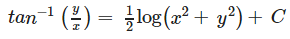# Test: Differential Equations (CBSE Level) - 1

## 25 Questions MCQ Test Mathematics (Maths) Class 12 | Test: Differential Equations (CBSE Level) - 1

Description
This mock test of Test: Differential Equations (CBSE Level) - 1 for JEE helps you for every JEE entrance exam. This contains 25 Multiple Choice Questions for JEE Test: Differential Equations (CBSE Level) - 1 (mcq) to study with solutions a complete question bank. The solved questions answers in this Test: Differential Equations (CBSE Level) - 1 quiz give you a good mix of easy questions and tough questions. JEE students definitely take this Test: Differential Equations (CBSE Level) - 1 exercise for a better result in the exam. You can find other Test: Differential Equations (CBSE Level) - 1 extra questions, long questions & short questions for JEE on EduRev as well by searching above.
QUESTION: 1

### Differential equations are equations containing functions y = f(x), g(x) and

Solution:

Differential equations are equations containing functions y = f(x), g(x) and derivatives of y with respect to x.

QUESTION: 2

### General solution of a given differential equation

Solution:

General solution of a given differential equation contains arbitrary constants depending on the order of the differential equation.

QUESTION: 3

### Find a particular solution of cos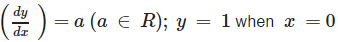Solution: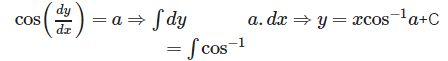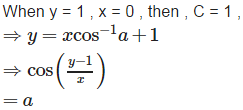QUESTION: 4

Differential equation of the family of circles touching the y-axis at origin is

Solution: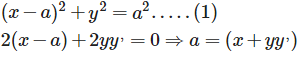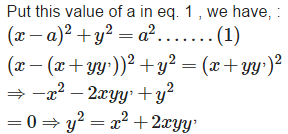QUESTION: 5

Which of the following is a homogeneous differential equation?

Solution:

y2dx + (x2− xy − y2) dy = 0 is a homogeneous differential equation ,because the degree of each individual term is same i.e. 2.

QUESTION: 6

Order of a differential equation is defined as

Solution:

Order of a differential equation is defined asthe order of the highest order derivative ofthe dependent variable.

QUESTION: 7

Particular solution of a given differential equation

Solution:

Particular solution of a given differential equationdoes not contain arbitrary constants i.e. a,b ,c etc.

QUESTION: 8

Find a particular solution of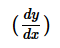= y tanx; y = 1 when x = 0

Solution: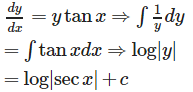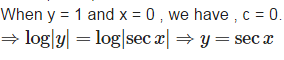QUESTION: 9

Differential equation of the family of parabolas having vertex at origin and axis along positive y-axis is

Solution: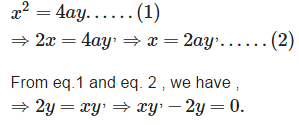QUESTION: 10

A first order linear differential equation. Is a differential equation of the form

Solution:

A first order linear differential equation. Is a differential equation of the form+ Py = Q or+ Px = Q

QUESTION: 11

Degree of a differential equation, when the equation is polynomial equation in y′ is

Solution:

Degree of a differential equation, when the equation is polynomial equation in y′ isHighest power (positive integral index) of the highest order derivative in the given differential equation.

QUESTION: 12

The number of arbitrary constants in the general solution of a differential equation of fourth order are:

Solution:

4 , because the no. of arbitrary constants is equal to order of the differential equation.

QUESTION: 13

For the differential equation xy= (x+2) (y+2) find the solution curve passing through the point (1, –1).

Solution: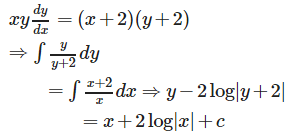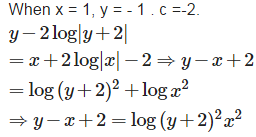QUESTION: 14

Differential equation of the family of ellipses having foci on y-axis and centre at origin is

Solution: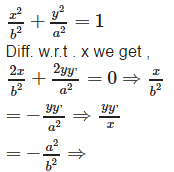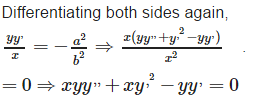QUESTION: 15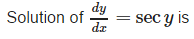Solution: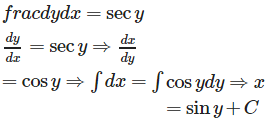QUESTION: 16

The order of the equation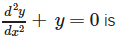Solution:

2

QUESTION: 17

The number of arbitrary constants in the particular solution of a differential equation of third order are:

Solution:

0 , because the particular solution is free from arbitrary constants.

QUESTION: 18

Find the particular solution of the differential equation log= 3x+4y, given that y = 0 and x = 0.

Solution: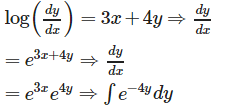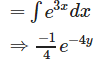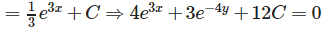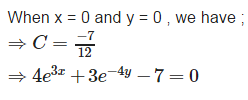QUESTION: 19

Find the equation of a curve passing through the point (0, 0) and whose differential equation is y′ = ex sin x.

Solution: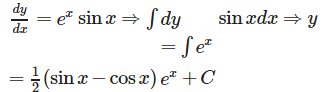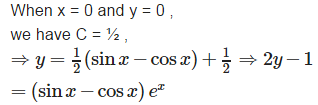QUESTION: 20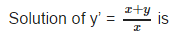Solution: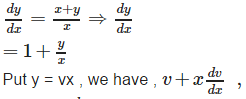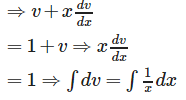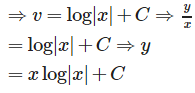QUESTION: 21

The order of the equation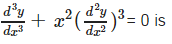Solution:

3

QUESTION: 22

General solution of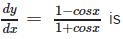Solution: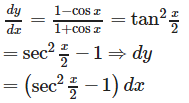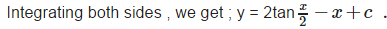QUESTION: 23

In a bank, principal increases continuously at the rate of r% per year. Find the value of r if Rs 100 double itself in 10 years (loge2 = 0.6931).

Solution:

Let P be the principal at any time t. then,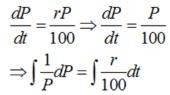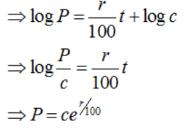When P = 100 and t = 0., then, c = 100, therefore, we have: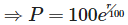Now, let t = T, when P = 100., then;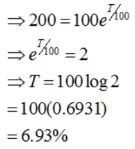QUESTION: 24

Find the equation of a curve passing through the point (0, –2) given that at any point (x, y) on the curve, the product of the slope of its tangent and y coordinate of the point is equal to the x coordinate of the point.

Solution: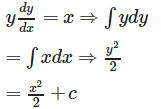When x= 0 and y = - 2 ,we have : c = 2,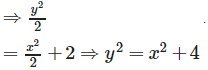QUESTION: 25

Solution of (x – y) dy – (x + y) dx = 0 is

Solution: